# Converse (logic) facts for kids

Kids Encyclopedia Facts

In mathematics and logic, a converse is a variant of an implication. More specifically, given an implication of the form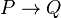$P \to Q$, the converse is the statement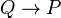$Q \to P$.

While a converse is similar to its originating implication, they are not logically equivalent. This means that the truth of an implication does not guarantee the truth of its converse (and vice versa).

As a logical connective, the converse of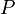$P$ and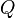$Q$ can be represented by the symbol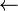$\leftarrow$ (as in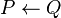$P \leftarrow Q$).

## Related pages

• Contrapositive

## Images for kidsConverse (logic) Facts for Kids. Kiddle Encyclopedia.